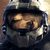0
Not a bug

# subroutine precision

dattorro 1 month ago updated by Pavel Holoborodko 1 month ago

"If input parameters a and b are multiprecision type, then all subsequent calculations inside the function will be conducted with high precision,..."

Re: Matlab 2021a under Windows 10:
User-written subroutines are executed in quadruple precision (34 digits) regardless of number of digits possessed by arguments passed to the subroutine.

For example: If arg1 has 100-digit precision and is passed to subroutine1(arg1), then computations within subroutine1 will be performed in 34-digit precision unless the following statement appears inside:

mp.Digits(mp.Digits(arg1));Not a bugWe do not recommend to mix different precisions. That is because sematic of mixed-precision computations are not well posed/defined. Do we have to use maximum precision of two arguments, or minimum, or average or else? That is a long-standing and non-resolved question in scientific community.

If you want to compare different precisions, then do all computations using one precision, store results for each precision (mat/txt file). And finally use the highest precision to load and compare them.

For example, assuming B>A:

Setup mp.Digits(A), run all the computations and store final results for precision A. Then setup mp.Digits(B) and re-run all computations from the start and store results for precision B. Setup mp.Digits(B), load all the results from files, and compare results (do not re-run the computations).

"User-written subroutines are executed in quadruple precision (34 digits) regardless of number of digits possessed by arguments passed to the subroutine."

Yes, this is true, if all expressions in subroutine use only arguments, e.g. r = a+b.

But, if expressions use some constants, e.g. r = sin(mp('pi')*x) - the final precision will be different. Because toolbox uses default precision to convert entities of "unknown" precision, like double numbers. So that if your default precision is mp.Digits(34), then it will be used for mp('pi'), regardless of what precision x has.